MORE IN Engineering Mechanics (EM)
SPPU First Year Engineering (Semester 2)
Engineering Mechanics (EM)
May 2014
Total marks: --
Total time: --
INSTRUCTIONS
(1) Assume appropriate data and state your reasons
(2) Marks are given to the right of every question
(3) Draw neat diagrams wherever necessary

Solve any qustion from Q1 & Q2
1 (a) The angle between the two concurrent forces is 90° and their resultant is 2500 N. The resultant makes an angle of 46° with one of the force, determine the magnitude of each force.
4 M
1 (b) A paricle moves along a straight line with an acceleration a=(4t2-2); where a is in m/s2 and t is in s. When t=0, the particle is at 2 m to the left of origin and when t=2 s the particle is at 20 m to left of origin. Determine the position of particles at t=4s.
4 M
1 (c) A cricket ball shot by a batsman from a height of 1.8 m at an angle of 30° with the horizontal with a velocity of 18 m/s is caught by a fielder at a height of 0.6 m from the ground. Determine the distance between the batsman and fielder.
4 M
1 (d) A block weighing 200 N is pulled up a 30° plane by a force P producing a velocity of 5 m/s in 5 s. If the coefficient of friction is 0.2, determine the magnitude of force P using impulse momentum principle.
4 M

2 (a) A thin rod is bend into a shape OABCD as shown in Fig 2 a. Determine the centroid of the bent rod with respect to origin O.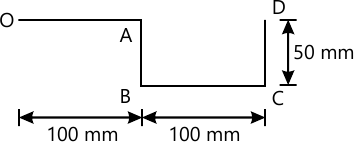4 M
2 (b) wo trains P and Q are 190 m and 160 m long moving in opposite directions on parallel tracks. The velocity of the shorter train is three times that of larger one. If the trains take 5 s to pass each other, determine the velocity of each train.
4 M
2 (c) Two blocks shown in Fig. 2 c have weight 90 N and 45 N. The coefficient of friction between the block A and the horizontal plane is 0.25. If the system is released from rest and the block B moves through a vertical distance of 0.6 m, determine the velocity of the blocks neglecting the friction in the pulley.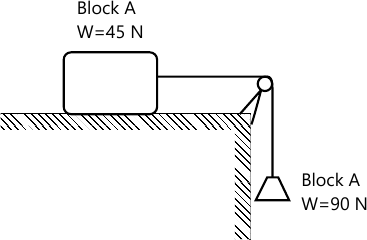4 M
2 (d) Determine the work done by all forces acting on the block of 18 kg as shown in Fig. 2 d as it moves 12 m upwards along the plane. Take coefficient of kineticfriction as 0.2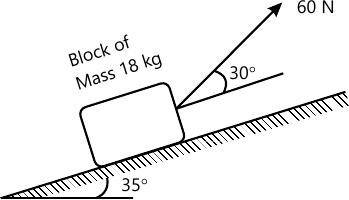4 M

3 (a) Find the angle of tilt ? with the horizontal so that the contact force at B will be one-half that at A for thesmooth cylinder. Refer Fig. 3 a.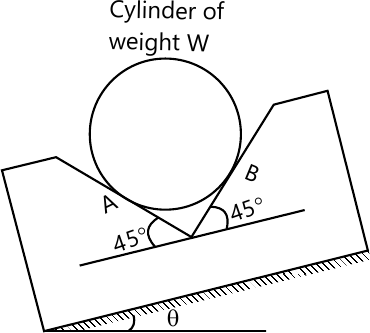6 M
3 (b) A 10 kN platform is supported vertically at A, B and C as shown in Fig. 3 b. If it carries a load of 6 kN, Determine the reaction at each support.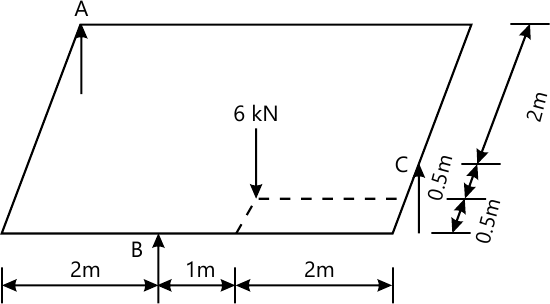6 M
3 (c) Determine the support reaction for the beam loaded and supported as shown in Fig. 3 c. 50 kN force is inclined at 30° to horizontal.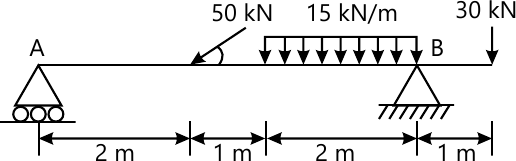5 M

4 (a) Three cables are joined at the junction C as shown inFig. 4 a. Determine the tension in cable AC and BC caused by the weight of the 30 kg cylinder.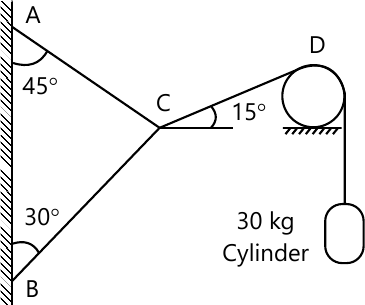5 M
4 (b) The cable exert force FAB=100 N and FAC=120 N on the ring at A as shown in Fig. 4 b. Determine the magnitude of the resultant force acting at A.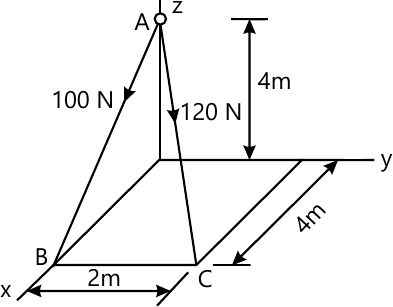6 M
4 (c) The uniform 18 kg bar OA is held in position as shown in Fig. 4 c by the smooth pin at O and the cable AB. Determine the tension in the cable and the reaction at O.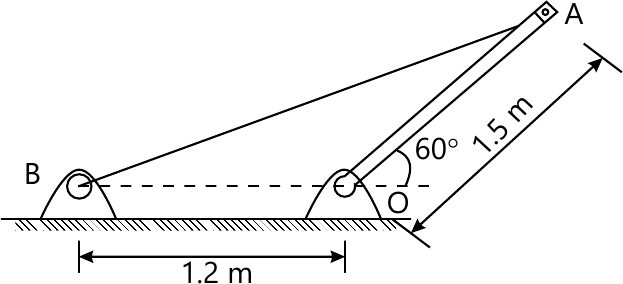6 M

5 (a) Determine the axial forces in each member of the plane truss as shown in Fig. 5 a.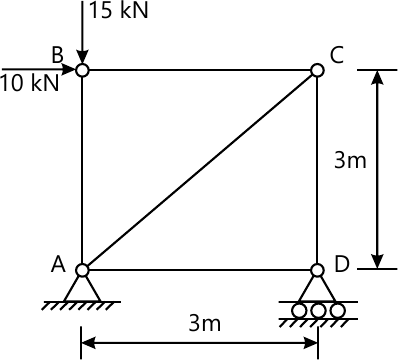6 M
5 (b) A body of weight 50 N is traveled along a rough horizontal plane by a pull of 18 N acting at an angle of 14° with the horizontal find the coefficient of staticFriction.
6 M
5 (c) Explain in brief angle of friction, coefficient of friction and laws of static friction.
5 M

6 (a) A cable supported at A and D at the same level over a span of 30 m is loaded as shown in Fig. 6 a. Determine the maximum tension in the segment of the cable.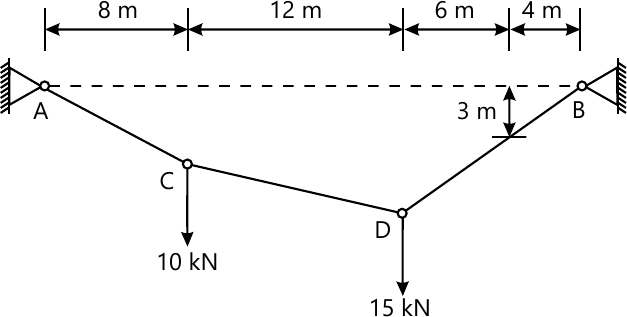6 M
6 (b) Determine the range of cylinder weight W as shown inFig. 6 b for which the system is in equilibrium. The coefficient of friction between cord and cylindrical support surface is 0.3 and that between100 N block and the incline surface is zero.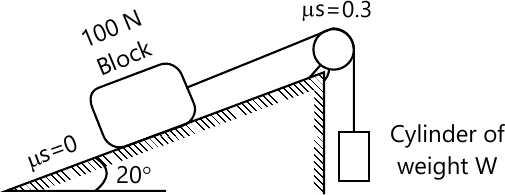6 M
6 (c) i. State the assumption for the analysis of plane truss.
ii. Define deficient, perfect and redundant truss
5 M

More question papers from Engineering Mechanics (EM)